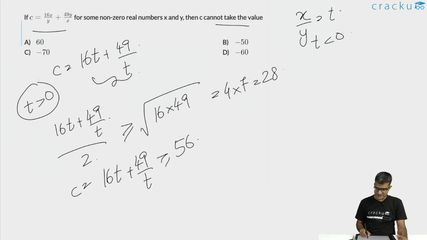Question 45

# If $$c=\frac{16x}{y}+\frac{49y}{x}$$ for some non-zero real numbers x and y, then c cannot take the value

Solution

Let $$\frac{x}{y}\ be\ t$$

Therefore, $$c=16t\ +\ \frac{49}{t}$$

Applying AM>= GM

$$\frac{\left(16t\ +\ \frac{49}{t}\right)}{2}\ge\ \left(16t\times\frac{49}{t}\right)^{\frac{1}{2}}$$

$$16t\ +\ \frac{49}{t}\ge56$$

When t is positive then c is greater than equal to 56.

When t is negative then c is less than equal to -56.

Therefore $$c\ \in\ \left(-\infty,\ -56\right]\ ∪\ \left[56,\infty\ \right]$$

As -50 is not in the range of c so it is the answer

### View Video Solution• All Quant CAT Formulas and shortcuts PDF
• 30+ CAT previous papers with solutions PDF

##### Suriya Narayanan

1 month ago

Why we are applying the concept of AM>GM?

1 year ago

B